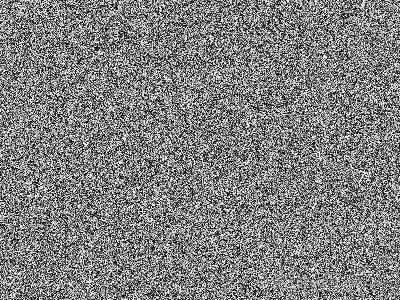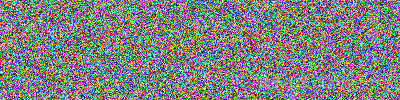#python-opencv学习笔记（二）

0
0
01. 云栖社区>
2. 博客>
3. 正文

## python-opencv学习笔记（二）

night李 2018-02-19 16:54:04 浏览1406

byteArray=bytearray(image)

bytearray含有恰当顺序的字节，可以通过显式转换和重构，得到numpy.array形式的图像：

grayImage=numpy.array(grayByteArray).reshape(height,width)
bgrImage=numpy.array(bgrByteArray).reshape(height,width,3)

import cv2
import numpy as np
import os

randomByteArray=bytearray(os.urandom(120000))
flatNumpyArray=np.array(randomByteArray)

grayImage=flatNumpyArray.reshape(300,400)
cv2.imwrite('RandomGray.png',grayImage)

bgrImage=flatNumpyArray.reshape(100,400,3)
cv2.imwrite('RandomColor.png',bgrImage)y.array结构针对数组操作有很好地优化，允许（bulk）操作。.array操作再OpenCV图像处理中会很方便使用，如对BGR图像的某一像素点进行操作，转换为白色像素点：

import cv2
import numpy as np
img[0,0]=[255,255,255]
cv2.imshow('1',img)
cv2.waitKey()

import cv2
import numpy as np
print(img.item(150,120,0))
img.itemset((150,120,0),255)
print(img.item((150,120,0)))

import cv2
import numpy as np
img[:,:,1]=0
cv2.imshow('1',img)
cv2.waitKey()

import cv2
import numpy as np
my_roi=img[0:100,0:100]
img[300:400,300:400]=my_roi
cv2.imshow('1',img)
cv2.waitKey()

numpy.array获取图像的属性：

import cv2
import numpy as np
print(img.shape)
print(img.size)
print(img.dtype)

(1080, 1920, 3)
6220800
uint8

Shape：NumPy返回包含宽度，高度和通道数的数组，在调试图像类型调试时有用，如果图像是单色或灰度的，将不包含通道值。
Size：该属性是指图像像素的大小
Dtype：该属性会得到图像的数据类型（通常为一个无符号整数型的变量和该类型占的位数，比如uint8类型）night李
+ 关注